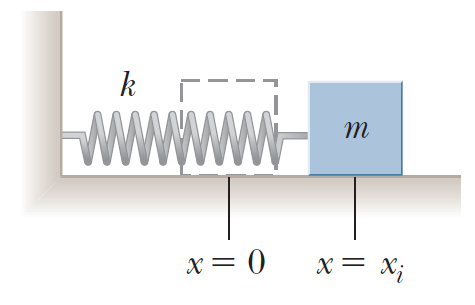Problem: A block of mass m = 2.00 kg is attached to a spring of force constant k = 500 N/m as shown in Figure P8.15. The block is pulled to a position xi = 5.00 cm to the right of equilibrium and released from rest. Find the speed the block has as it passes through equilibrium if(a) the horizontal surface is frictionless and(b) the coefficient of friction between block and surface is µk = 0.350.

FREE Expert Solution
90% (321 ratings)
FREE Expert Solution

In this problem, we'll apply energy conservation to determine the velocity of the mass at the equilibrium position.

Spring potential energy:

$\overline{){{\mathbf{U}}}_{{\mathbf{s}}}{\mathbf{=}}\frac{\mathbf{1}}{\mathbf{2}}{\mathbf{k}}{{\mathbf{x}}}^{{\mathbf{2}}}}$

90% (321 ratings)
Problem Details

A block of mass m = 2.00 kg is attached to a spring of force constant k = 500 N/m as shown in Figure P8.15. The block is pulled to a position xi = 5.00 cm to the right of equilibrium and released from rest. Find the speed the block has as it passes through equilibrium if

(a) the horizontal surface is frictionless and
(b) the coefficient of friction between block and surface is µk = 0.350.